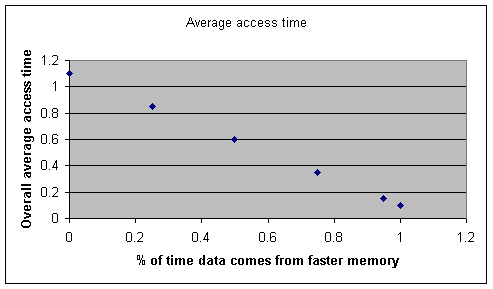Caching: A Set-up for Getting Best-possible Memory Access Times

Imagine you have 2 kinds of memory: fast and slow. They have the following access times.

Fast memory: 0.1 time units
Slow memory: 1.0 time units

You design a computer so that it can read something that's in the fast memory directly. To read something in the slow memory it has to be transferred to fast memory, then read from there. So retrieving something that sits in the slow memory takes approximately the combined time for getting it out of slow, then getting it out of fast, or 1.0 + 0.1 = 1.1 units. Retrieving stuff out of fast memory takes only 0.1 units.

So the effective access times are:

Fast memory: 0.1 time units
Slow memory: 1.1 time units

If you accessed memory many times, again and again, suppose your target data happened to lie in the 2 types of memory on a 50/50 basis. Half the time, getting your data would take 1.1 time units; the other half only 0.1 units. So the average access time would work out to

(0.50 x 1.1) + (0.50 x 0.1) = 0.60

That's right about in the middle as you would expect. On the other hand, wouldn't it be great if you could somehow engineer the advantage of having the data you wanted positioned, at the time you wanted it, in the fast memory 95% of the time? Then the average time to get your data would be only

(0.05 x 1.1) + (0.95 x 0.1) = 0.15

Other possibilities might be if to-be-retrieved data awaited you in the fast memory all the time, 75% of the time, 25% of the time, or never. For these and the above two cases the range of average retrieval times is

(0.00 x 1.1) + (1.00 x 0.10) = 0.1

(0.05 x 1.1) + (0.95 x 0.1) = 0.15

(0.25 x 1.1) + (0.75 x 0.1) = 0.35

(0.50 x 1.1) + (0.50 x 0.1) = 0.60

(0.75 x 1.1) + (0.25 x 0.1) = 0.85

(1.00 x 1.1) + (0.00 x 0.1) = 1.1

The more frequently you can arrange to have wanted data waiting for you in the fast place, the faster your machine's overall or global average access to data will be. The above 6 equations give us 6 points to graph, showing overall access time as a function of how much of the time your data is obtainable from the fast memory.Strategizing to predetermine where to-be-accessed data shall reside when needed, seeking to preposition it in the fastest memory available within the computer, is called caching. Doing it successfully raises the overall performance of a machine.

The percentage of time the data sought lies in the faster memory is called the "hit ratio." If you seek data 100 times and find it in the fast memory 80 times, the hit ratio would be 80%. If we call the hit ratio H, then the above equations can be generalized as

T = (1 - H) x 1.1 + H x 0.1

or

Taverage = (1 - H) x Tslow + H x Tfast

where T stands for access time.  You could rearrange this to express what the hit ratio is in terms of the 3 access times

H = (Tslow - Tave)/(Tslow - Tfast)

So we can achieve far better-than-expected access times if only we can figure out how to pre-position the data we're going to want (a crystal ball to predict the future would help here) in the fast memory shortly before we want it. We don't have a crystal ball. Is there any other way to accomplish that?